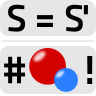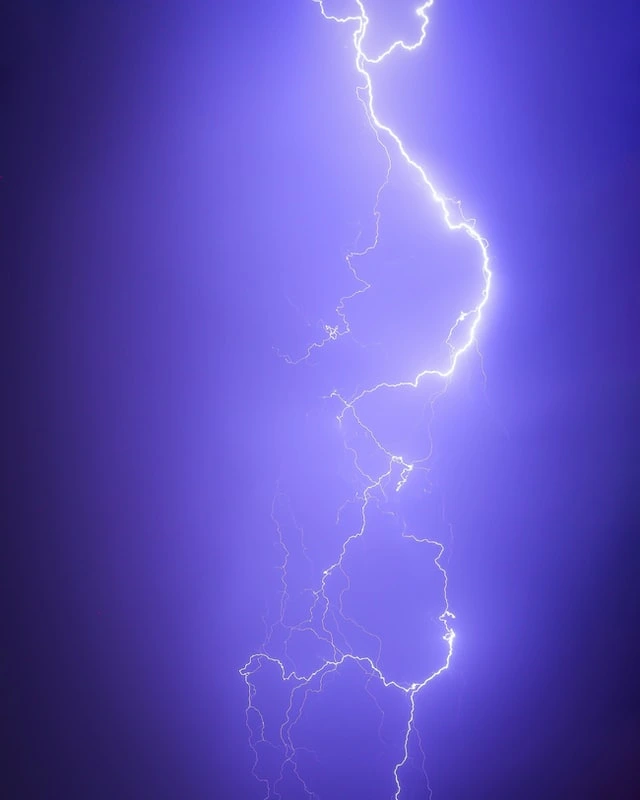Usually, gauge transformations are used to simplify certain calculations. In this problem, we want to use them to derive charge conservation from the invariance of the action S.

## Problem StatementLet us make ourselves familiar with the implications of gauge invariance. Usually we get to know the gauge transformations as a technical trick. Nevertheless, there is much more behind it - following Noether's theorem, any transformation of the action implies a conserved current and charge as we already know from theoretical mechanics.

In electrodynamics, one example is the conservation of electric charge. Let us understand the principle in two steps:

1. Show that the electric and magnetic fields are invariant under the gauge transformationswhere λ(xμ) is an (almost) arbitrary scalar function.
2. Calculate the change of the action
$$S=\int\mathcal{L}\left(x^{\mu}\right)dVdt$$
with respect to an arbitrary gauge transformation. The condition S = S' results in a well known condition you may use to derive charge conservation.

Bonus: Explain a relation of lightning to gauge invariance in electrodynamics. This is a more qualitative task. Hopefully you can think about this question a bit and you get a bit inspired how such seemingly mathematical topics are very interesting concepts.

Image credits go to Frankie Lopez!

## Hints

The Lagrangian of the electromagnetic field with present charges and currents can be expressed as

$\begin{eqnarray*} \mathcal{L}\left(x^{\mu}\right)&=&\frac{\varepsilon_{0}}{2}\mathbf{E}\left(x^{\mu}\right)^{2}-\frac{1}{2\mu_{0}}\mathbf{B}\left(x^{\mu}\right)^{2}\\&&-\phi\left(x^{\mu}\right)\rho\left(x^{\mu}\right)+\mathbf{A}\left(x^{\mu}\right)\cdot\mathbf{j}\left(x^{\mu}\right)\ . \end{eqnarray*}$

The coupling of the charges and currents is naturally related to the potentials. You may want to express the Lagrangian in terms of the potentials alone for the given problem.

So, let's use the hints and find some nice gauge invariance implications!

## Gauge Transformations do not Change the Field Equations

Let us make ourselves first a little familiar with gauge transformation calculations by confirming that Maxwell's equations are invariant under such transformations. Again, the gauge transformations (as proclaimed) are given by

$\begin{eqnarray*} \phi\left(x^{\mu}\right)\rightarrow\phi^{\prime}\left(x^{\mu}\right)&=&\phi\left(x^{\mu}\right)-\partial_{t}\lambda\left(x^{\mu}\right)\ \text{and}\\\mathbf{A}\left(x^{\mu}\right)\rightarrow\mathbf{A}^{\prime}\left(x^{\mu}\right)&=&\mathbf{A}\left(x^{\mu}\right)+\nabla\lambda\left(x^{\mu}\right)\ . \end{eqnarray*}$

We now have to check, if the fields are invariant under such transformations given their relations to the potentials. First for the magnetic field:

$\begin{eqnarray*} \mathbf{B}^{\prime}\left(x^{\mu}\right)&=&\nabla\times\mathbf{A}^{\prime}\left(x^{\mu}\right)\\&=&\nabla\times\left[\mathbf{A}\left(x^{\mu}\right)+\nabla\lambda\left(x^{\mu}\right)\right]\\&=&\nabla\times\mathbf{A}\left(x^{\mu}\right)\\&=&\mathbf{B}\left(x^{\mu}\right)\quad\surd \end{eqnarray*}$

and now for the electric field:

$\begin{eqnarray*} \mathbf{E}^{\prime}\left(x^{\mu}\right)&=&-\nabla\phi^{\prime}\left(x^{\mu}\right)-\partial_{t}\mathbf{A}^{\prime}\left(x^{\mu}\right)\\&=&-\nabla\left[\phi\left(x^{\mu}\right)-\partial_{t}\lambda\left(x^{\mu}\right)\right]-\partial_{t}\left[\mathbf{A}\left(x^{\mu}\right)+\nabla\lambda\left(x^{\mu}\right)\right]\\&=&-\nabla\phi\left(x^{\mu}\right)-\partial_{t}\mathbf{A}\left(x^{\mu}\right)\\&=&\mathbf{E}\left(x^{\mu}\right)\quad\surd \end{eqnarray*}$

Note that we did not have to show that gauge transformations do not change Maxwell's equations if they do not have an impact on the fields.

Let us now find out how gauge invariance implies charge conservation.

## From Gauge Transformations to Charge Conservation

We know that that the free-space Lagrangian density in terms of the potentials is given by$\begin{eqnarray*} \mathcal{L}\left(x^{\mu}\right)&=&\frac{\varepsilon_{0}}{2}\left(\nabla\phi\left(x^{\mu}\right)+\partial_{t}\mathbf{A}\left(x^{\mu}\right)\right)^{2}-\frac{1}{2\mu_{0}}\left(\nabla\times\mathbf{A}\left(x^{\mu}\right)\right)^{2}\\&&-\phi\left(x^{\mu}\right)\rho\left(x^{\mu}\right)+\mathbf{A}\left(x^{\mu}\right)\cdot\mathbf{j}\left(x^{\mu}\right) \end{eqnarray*}$and the action is just its four-dimensional integral,$S = \int\mathcal{L}\left(x^{\mu}\right)dVdt\ .$From the Lagrangian formulation, Maxwell's equations can be derived by the requirement that the action is extremal, $$\delta S=0$$, which leads to the Euler-Lagrange equations. I particularly like $$\delta S=0$$, because it's the summation of all of physics that we know...

Nevertheless, since we know that the field equations are not changed by gauge transformations, this also implies that the action remains unchanged under such a transformation. To find the relation to charge conservation, we just have to relate the actions in the different gauges and look what is left. First, we express the transformed Lagrangian in the old gauge:

$\begin{eqnarray*} \mathcal{L}^{\prime}\left(x^{\mu}\right)&=&\frac{\varepsilon_{0}}{2}\left(\nabla\phi^{\prime}\left(x^{\mu}\right)+\partial_{t}\mathbf{A}^{\prime}\left(x^{\mu}\right)\right)^{2}-\frac{1}{2\mu_{0}}\left(\nabla\times\mathbf{A}^{\prime}\left(x^{\mu}\right)\right)^{2}\\&=&-\phi^{\prime}\left(x^{\mu}\right)\rho\left(x^{\mu}\right)+\mathbf{A}^{\prime}\left(x^{\mu}\right)\cdot\mathbf{j}\left(x^{\mu}\right)\\&=&\frac{\varepsilon_{0}}{2}\left(\nabla\left[\phi\left(x^{\mu}\right)-\partial_{t}\lambda\left(x^{\mu}\right)\right]+\partial_{t}\left[\mathbf{A}\left(x^{\mu}\right)+\nabla\lambda\left(x^{\mu}\right)\right]\right)^{2}\\&&-\frac{1}{2\mu_{0}}\left(\nabla\times\left[\mathbf{A}\left(x^{\mu}\right)+\nabla\lambda\left(x^{\mu}\right)\right]\right)^{2}\\&&-\left(\phi\left(x^{\mu}\right)-\partial_{t}\lambda\left(x^{\mu}\right)\right)\rho\left(x^{\mu}\right)+\left[\mathbf{A}\left(x^{\mu}\right)+\nabla\lambda\left(x^{\mu}\right)\right]\cdot\mathbf{j}\left(x^{\mu}\right)\ . \end{eqnarray*}$

Ok, this step was more like copy and paste, but we see directly that a lot of terms cancel and we are left with

$\begin{eqnarray*} \mathcal{L}^{\prime}\left(x^{\mu}\right)&=&\frac{\varepsilon_{0}}{2}\left(\nabla\phi\left(x^{\mu}\right)+\partial_{t}\mathbf{A}\left(x^{\mu}\right)\right)^{2}-\frac{1}{2\mu_{0}}\left(\nabla\times\mathbf{A}\left(x^{\mu}\right)\right)^{2}\\&&-\phi\left(x^{\mu}\right)\rho\left(x^{\mu}\right)+\mathbf{A}\left(x^{\mu}\right)\cdot\mathbf{j}\left(x^{\mu}\right)\\&&+\partial_{t}\lambda\left(x^{\mu}\right)\rho\left(x^{\mu}\right)+\nabla\lambda\left(x^{\mu}\right)\cdot\mathbf{j}\left(x^{\mu}\right)\\&=&\mathcal{L}\left(x^{\mu}\right)+\partial_{t}\lambda\left(x^{\mu}\right)\rho\left(x^{\mu}\right)+\nabla\lambda\left(x^{\mu}\right)\cdot\mathbf{j}\left(x^{\mu}\right)\ . \end{eqnarray*}$

The last two terms look already very familiar - they remind us of the continuity equation, but how can we make this relation obvious? We have to remember that we are talking from the perspective of the action, $$S=\int\mathcal{L}d^{4}x$$ and that this is the invariant quantity.

With our result, we can calculate the difference between actions under a general gauge transformation:

$\begin{eqnarray*} S^{\prime}-S&=&\int\partial_{t}\lambda\left(x^{\mu}\right)\rho\left(x^{\mu}\right)+\nabla\lambda\left(x^{\mu}\right)\cdot\mathbf{j}\left(x^{\mu}\right)dVdt\ . \end{eqnarray*}$

The point is now, that the action cannot change under gauge transformations! Remember, $$S$$ is a physical quantity whereas gauge transformations are merely and adjustment of the potentials. Using partial integration, we find

$\begin{eqnarray*} 0\overset{!}{=}S^{\prime}-S&=&-\int\lambda\left(x^{\mu}\right)\left[\partial_{t}\rho\left(x^{\mu}\right)+\nabla\mathbf{j}\left(x^{\mu}\right)\right]dVdt \end{eqnarray*}$

which is really just the continuity equation - derived from the gauge invariance of the action!

Of course, the continuity equation now implies charge conservation, since

$\begin{eqnarray*} \frac{d}{dt}\int\partial_{t}\rho\left(x^{\mu}\right)dVdt&=&\frac{d}{dt}\int\rho\left(x^{\mu}\right)dV\\&=&-\int\nabla\mathbf{j}\left(x^{\mu}\right)dV\\&=&-\int\mathbf{j}\left(x^{\mu}\right)\cdot d\mathbf{A}=0 \end{eqnarray*}$

if the current density is localized, or has a compact support as we may naturally assume.

Our little calcuation is really just an application of Noether's theorem from which we could have directly reasoned the continuity equation $$\partial_{\mu}j^{\mu}=0$$.

## Relation of Gauge Invariance to Lightning

You might have asked yourself: what was the motivation for this somewhat qualitative task? Well, I hope it made you think a bit about lightning as an electromagnetic phenomenon.

Lightning itself can be described as electrostatic discharge between the atmosphere or between clouds. The sudden charge exchange leads to an enourmous amount of released energy, which is in the GJ (Gigajoule) region. That is an enourmous amount of energy, which, for example leads to a lot of radiation.

Very impressive is the speed of electrons inside a lightning bolt. Electrons reach about 300,000 km/h, that's around 200,000 m/h according to National Geographic. That's around 80km/s, a relativistic speed. Remember that satellites in low earth orbit travel at around 10 km/h. And they have to take care of relativistic effects.

Gauge invariance now tells us two things in this situation:

1. Charge is conserved, no matter the energy or the speed or whatever
2. The electromagnetic potentials can be changed by gauge transformations. Even the electric fields can be changed by coordinate transformations (think about moving with the electrons). However, what is not changed, in any gauge or coordinate system, is the physics. Such, say, mathematical adjustments do not alter what is actually happening to the physical world!

Just take some one or two minutes and think about how awesome these statements actually are.